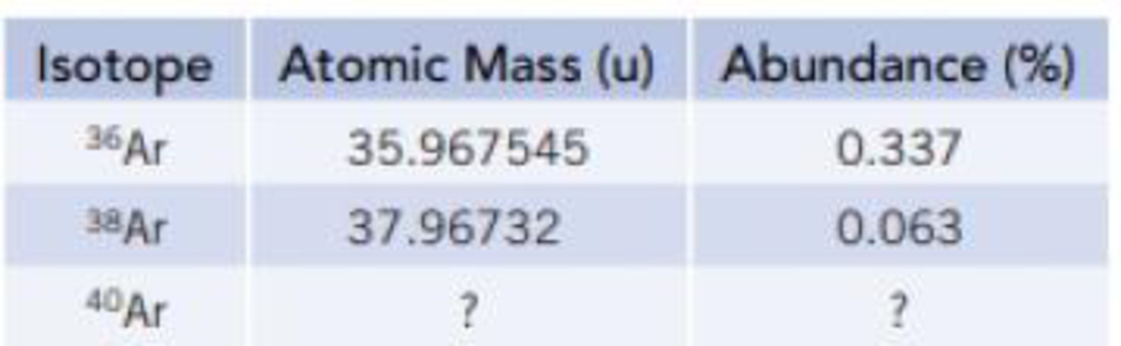Chapter 2.8, Problem 3.3ACP

Chapter
Section
Textbook Problem

Atmospheric argon is a mixture of three stable isotopes. 36Ar. 38Ar, and 40Ar. Use the information in the table below to determine the atomic mass and natural abundance of 40Ar.Interpretation Introduction

Interpretation: The atomic mass and natural abundance for given 40Ar has to be determined from the given data’s of other isotopes.

Concept Introduction:

The average mass of argon is 39.948 u.

Equation for average weight is,

average mass=(%abundanceisotop-1100)×(massofisotop-1) +(%abundanceisotop-2100)×(massofisotop-2)+etc

Two species are said to be isotopes if they have same atomic number but different mass number.

Explanation

There are three isotopes for argon atom.

The mass of isotope-1 is 35.967545u and its abundance is 0.337%.

The mass of isotope-2 is 37.96732u and its abundance is 0.063%.

Therefore, abundance of isotope-3 is 100%-(0.337%+0.063%)=99.6%

Equation for average weight is,

average weight=(%abundanceisotop-1100)×(massofisotop-1) +(%abundanceisotop-2100)×(massofisotop-2)+etc

Therefore,

average weightofargon=(0.337100)×(35

Still sussing out bartleby?

Check out a sample textbook solution.

See a sample solution

The Solution to Your Study Problems

Bartleby provides explanations to thousands of textbook problems written by our experts, many with advanced degrees!

Get Started